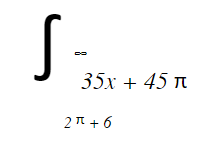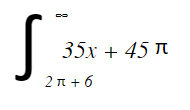# Easy way to represent math by a few lines of HTML via CSS.

• By Quickcard
• Last update: Oct 28, 2022

# MathCSS

A verbose, responsive, and easy way to represent basic math and calculus by a few lines of HTML without the need for a heavier JavaScript library. Built exclusively in CSS using a block-chain technique. Sister library to: https://github.com/mathexl/chemistry-cssNew in 2.5.0

• Added support for display in white

What's MathCSS good for?

• Quick depictions of integrals, summations, products, and alike.
• Scalable, responsive design. MathCSS is built like a choo-choo-s train.
• Special math symbols without looking up the unicode.

Support soon to be added for:

• Multi-bounds

## Usage

### Getting Started

">
<link href="path/to/math.css" rel="stylesheet">

And you're ready to go! Documentation is easy as provided below. Simply, add an equation attribute to begin as follows (you can use the mathbox alias as well):

<div equation>

div>

If you want the display to be entirely in white, add the class white to the

tag. So:

">
<div equation class="white">

div>

### Integrals, Products, Summations

#### The goal of MathCSS is so that your HTML reads like math. You can easily add integral, doubleintegral, tripleintegral, product, summation like such:

<div equation>
<div integral>

div>
div>

To specify bounds and input, simply use upperbound, lowerbound, and of attributes:

<div integral>
<div upperbound>
5x
div>
<div lowerbound>
39x
div>
<div of>
35x + 45
div>
div>

upperbound, lowerbound, and of will only display correctly when inside integral, doubleintegral, tripleintegral, product, or summation.

Since upperbound and lowerbound attributes are absolute, the order in which you declare them doesn't matter.

### Fractions, Derivatives (a special fraction), Partial Derivatives

#### MathCSS has built-in support for fraction, derivative (short fraction), and partial derivative.

<div equation>
<div fraction>

div>
div>

To specify top and bottom, simply use top and bottom attributes:

<div fraction>
<div top>
5x
div>
<div bottom>
39x
div>
div>

While MathCSS cannot support infinite fraction's within each other, it can go down a scope of 2. You can embed a subfraction in a fraction, but not a subfraction in a subfraction in a subfraction due to sizing constraints. For instace, the following code will work:

<div fraction>
<div top>

<div fraction>
<div top>
35y + 4x
div>
<div bottom>
12x + 4z
div>
div>

+ y<sup>2sup> +

<div fraction>
<div top>
35x + 4y
div>
<div bottom>
12z + 4
div> div> div> <div bottom> <div fraction> <div top> 35x + 2x div> <div bottom> 11x + 4 div> div> + 5 + <div fraction> <div top> 35x + 4x div> <div bottom> 12x + 4 div> div> div> div>

If you ever need to enclose a fraction in a fraction in a fraction, it is optimal to use a de facto (x / y) notation - that helps with readibility anyway due the decreasing sizes of integer.

Since derivatives are technically both a fraction and an operand, they behave like a fraction in MathCSS. For the term that is being derived, just follow the derivative with a term tag. For example:

<div derivative>
<div top>
x
div>
<div bottom>
y
div>
div>

<div term>
35x + 45
div>

MathCSS also tries to allow users to never have to look up the unicode for common math symbols. Hence, there is a built in partial derivative function, just exclude the special d's. And yup, partial derivative reads just like English, use two words, not one or hyphenated.

<div partial derivative>
<div top>
x
div>
<div bottom>
y
div>
div>

<div term>
35x + 45
div>

### Limits

#### Creating limits involves just three parts, the variable variable, what it is approaching goingto, and the term represented by of. The following code below would render the limit of x approaching infinity of thirty-five x squared plus twelve x plus nine.

<div limit>
<div variable>
x
div>
<div goingto>
<hr infty>
div>
<div of>
35x<sup>2sup> + 12x + 9
div>
div>

### Square Roots and Roots

#### You can add square roots easily using the root attribute. You can also specify the degree of the root with degree. Use of for the term. The degree tag is optional.

<div root>
<div of>
35x + 45y<sup>2sup> + 23
div>
<div degree>
4
div>
div>

### Vector Brackets

#### You can add vector wide brackets using vector instead of term.

<div vector>
4x, 3
div>

### HR Magic!

#### Using HTML's built in   tag, we can easily add common math characters into our equation without the messy closing tags.

For example, if you want to show the integral from the upperbound of infinity to the lower bound of 2 pi, of 35x + 45, simply:

<div integral>
<div upperbound>
<hr infty>
div>
<div lowerbound>
2<hr pi> + 6
div>
<div of>
35x + 45<hr pi>
div>
div>

The above code renders:The tags

and   will automatically show. The available subclasses range from greek commons, discrete math symbols, and common figures. See the full list below. Simply use   -- no necessary.

Operand and Values:

partial, pm, infty, approx, neq, leq, geq

Discrete Math:

forall, exists, nexists, in, notin, and, or, cap, cup, congruent, subsetleft, subsetright, notsubsetleft, notsubsetright, subsetorequaltoright, subsetorequaltoleft, notsubsetorequaltoleft, notsubsetorequaltoright

Greek Letters:

pi, alpha, beta, lambda, delta (more coming soon)

### Absolute Value

To add absolute value, you can either use the u tag or use with whatever is being absoluted. For instance:

HTML -5 + -5 = 10


### General Operands

If you need to add, subtract, multiply, or divide two terms in a sequence, use add, subtract, multiply, divide.

Note that these attributes will only display functionally within the general equation tag and not within a integral for spacing reasons.

For example:

HTML

5x2

39x

35x + 45

5y

3y

35y78 + 45



### Exponents

If you want to add exponents, use the standard HTML tags:

<div integral>
<div upperbound>
5x<sup>2sup>
div>
<div lowerbound>
39x
div>
<div of>
35x + 45
div>
div>

### Matrixes

The inherent complexity of matrixes makes them a bit different syntactically in Math.css. Math.css supports matrixes up to the size of 8 rows and infinite (within reason) cols. First, you need to declare the type of matrix along with the number of rows.
Due to the limits of CSS, the amount of rows needs to be statically declared unlike the number of cols.

<div matrix two>

div>

This will create a matrix of two rows. The key attributes three, four, five, six, seven, eight all work respectively.

Due to the need to dynamically size the width of entries for long entries, we need to insert things into a matrix on a column to column basis.

To make things easy, math.css ships with two different ways of inserting columns. The first is the b-a method, which encloses a tags (entries) with b tags (cols). For instance, if I wanted the matrix of two by two rows, with the first row being 6 and 12, and the second row being 4 and 5, we get this:

<div matrix two>
<b>
<a>6a>
<a>4a>
b>
<b>
<a>12a>
<a>5a>
b> div>

However, this can get tedious given the amalgam of tags necessary. Therefore, you can instead use the alternate

method where you separate each entry with an   tag. Therefore, nothing is enclosed.

<div matrix two>
<b>
6
<hr>
4
b>
<b>
12
<hr>
5
b>
div>

### Choose

If you want to add probability constructs like n choose k, simply use the choose tag, similar to how you would construct a fraction. Using the tags top and bottom for each part of the choose.

<div choose>
<div top>
4
div>
<div bottom>
3
div>
div>

MIT License: free to use and open source.

Want to add something? Feel free to fork or email me at [email protected]. Or even send me a tweet to @mathewpregasen :).

## Github

https://github.com/parsegon/math-css

• 1

### Convert non-TeX-friendly codes to TeX-y ones

e.g. notanelementof becomes notin, borrowing from LaTeX's \notin syntax.

Convenient because this means that we can guarantee that there is a TeX-esque typesetting scheme for any of these items, and there will never be naming conflicts (TeX figured those out for us already)!

• 2

### Thoughts for a parser

Alright. So this is overzealous, absolutely. But I'd love to have a super-basic parser that can convert something like \int^{x}_{y} dt to the correct HTML to be used in your markup.

As my proof of concept, try running the compile() function on a string like str = "\\int^{1}_{0} i dx". It returns the minified HTML,

<div integral><div upperbound>1</div><div lowerbound>0</div><div of>i dx</div></div>


If anyone is interested in talking about how better to implement a grammar, I'd love to chat. This is certainly not the right strategy, but I really really want "realtime" "TeX" "rendering" (note the heavy use of quotes) when I'm playing around in markdown or something.

• 3

### Is this the only web math solution that supports arbitrary HTML within math expressions?

If so, you might want to mention that in the readme. Also if so, thank you!!! I will need it and will send you a link once I have something to show. :-)

• 4

### Alignement of elements not defined

As I tried an example out of the box, I found, that elements were not aligned properly.Defining the line-height in div[equation] solves it:

  div[equation] {
box-sizing: border-box;
height: auto;
margin: 0px auto 0;
min-height: 100px;
width: 80%;
font-family: "stixgeneralregular";
line-height: 1.2;
}
`